﻿ The Fourier, Laplace Transformations and the Newton Potential

### The Fourier, Laplace Transformations and the Newton Potential

Andrey Pavlov V.OPEN ACCESSPEER-REVIEWED

## The Fourier, Laplace Transformations and the Newton Potential

Andrey Pavlov V.Higher Mathematics, Moscow Inst. of Rad., Electr. And Automat. (MIREA), pr. Vernadskogo, Moscow, Russia

### Abstract

With help of a methods of Fourier`s analysis we consider the analytic continuation of Laplace transformation (with help a values on the complex axis) to the left part of plane, when the continuation is odd ore even.

• V., Andrey Pavlov. "The Fourier, Laplace Transformations and the Newton Potential." American Journal of Applied Mathematics and Statistics 2.6 (2014): 398-401.
• V., A. P. (2014). The Fourier, Laplace Transformations and the Newton Potential. American Journal of Applied Mathematics and Statistics, 2(6), 398-401.
• V., Andrey Pavlov. "The Fourier, Laplace Transformations and the Newton Potential." American Journal of Applied Mathematics and Statistics 2, no. 6 (2014): 398-401.

 Import into BibTeX Import into EndNote Import into RefMan Import into RefWorks

### 1. Introduction

A communication between potentials of Newton and evenness of functions of type of Laplace transformations from Fourier transformations (the theorems 1, 3) is of principle for the article. The main result of the article are the theorems 1 and 2.

The second purpose of the article is a clarification of results of the works [1, 2] (the remarks 1, 2, 3 and the theorem 3).

The results look like were formulated in works of author ([1, 2, 3, 4]). In the works of author [1, 2] the specifying investigation (the remark 2) was absent, and in the works ([3, 4]) other methods were used.

We will mark, that unforeseeable results of theorem 2 and the theorems 3 are proved by different methods fully. A primary purpose of the article is a proof of these theorems.

A discussion of an consequences in the direction of possible compound of axiomatic notions of theory of functions of complex variable and the classic mathematical analysis is beyond the article.

### 2. The Laplace Transformation on the Complex Axis

Theorem 1.

The equalities

1.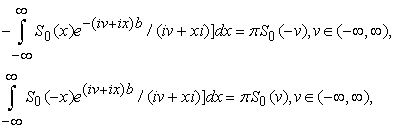2. for all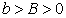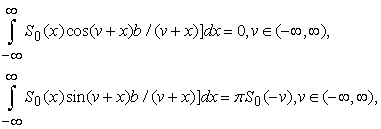take place, if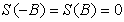, if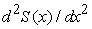is continuous on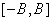, and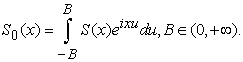Proof.

We use (it is well-known)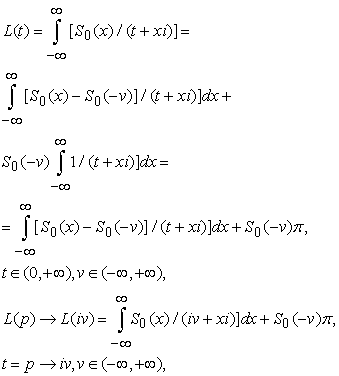where from remark 1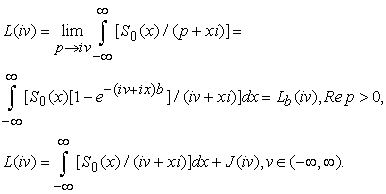(After replacement of variable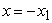and after the substitution in place of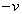value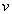we obtain the second part of the equality 1 in the theorem 1).

The complex and the real parts of the first equality are the second equalities of the theorem 1.

Remark 1.

In the conditions of the theorem 1 the equalities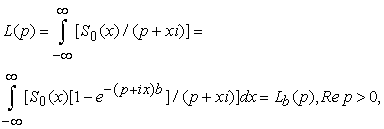take place.

Proof.

From the inversion formula of the Fourier transformation we get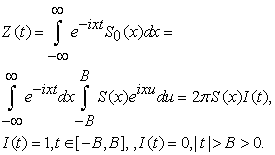We obtain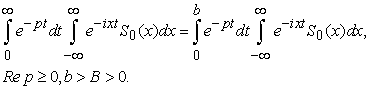From the conditionwe obtain all the conditions of the proposition 1 (taking into account the formula of integration on parts).

Proposition 1.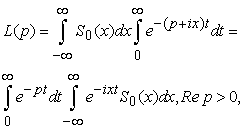if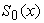is continuous for all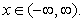By definition,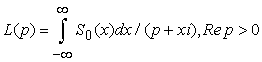.

(The proposition 1 takes place, if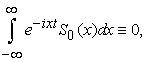for all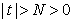; the functions are considered in the theorem 1).

Proof.

For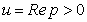from inequality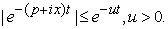we can change the limits of integration in the initial expression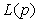().

The proposition 1 is proved.

After change of order of integration () from the proposition 1 we get the last part of the remark 1 :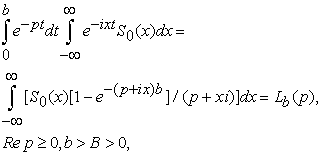and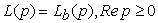.

We will prove the main theorem 2.

Theorem 2.

In the conditions of the theorem 1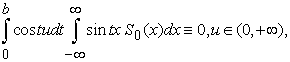Proof.

From the theorem 1 we have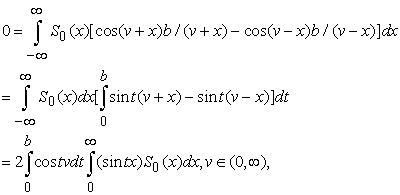for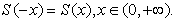The main theorem 2 is proved.

Now, we will prove the remark 2. The remark is absent in works of author ([1, 2]).

Remark 2.

In conditions of the remark 1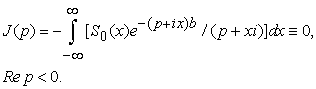Proof.

After the change of limits of integration (), we obtain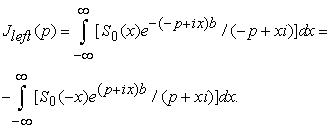Obviously, for the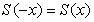function there is a limit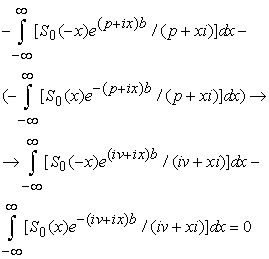where for the last equality we use the first part of the remark 3. We obtain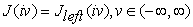, and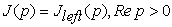too (), (the regularity of the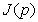function in an open area of imaginary axis ensues from the obvious regularity of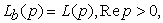in all complex plane).

We get.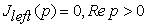from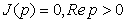. For the odd the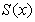function the remark 2 is proved.

For the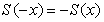function we repeat the same reasoning of the remark 3 but for the sum.

Consequence.

From the remark 2 as analytical continuation of thefunction (not as in the theorem 1, see the theorem 2 too) we get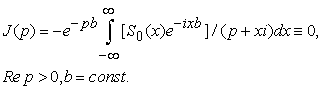Theorem 3

In the conditions of remark 1 to the lemma 1 we get, that the analytic continuation of function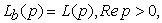from the right part of plane to left is equal to the analytic expression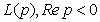.

Proof.

From the remark 2 we get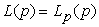not only for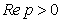, but for all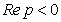. The existence of the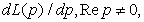is obvious (in the form). We can use ()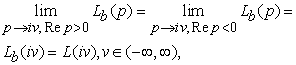and as in ([1, 2]) from the theorem about the analytic continuation of the functions continuous on border we obtain, thatis the same analytic function as forso as.

Remark 3. ([1, 2]).

The analytic expressionis even or odd expression, if the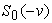function is odd or even accordingly. It is obviously, if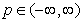. From the theorem 3 we obtain, that the analytic functionis even or odd in the all complex plain too.

Remark 4.

We will notice, that from the investigation of evenness ofin the area of imaginary axis, we obtain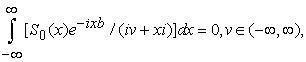which it follows from taking into account the remark 2 and the theorem 3, but from point of the convergence ofto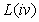, the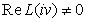, and from point of analytic continuations (physically from point of smooth transition-flow through the imaginary axis) the such actual values are absent.

The first given fact was marked by other methods in a few more general situation, but for other functions, in the works of author ([3, 4]).

### 3. Conclusion

The first unforeseeable result is the theorem 2 (see so the formula (5) on the 209 page in the work ).

The second unforeseeable result we obtain from the remark 2 and the theorem 3: from point of the convergence ofto, the(thevalue in the theorem 1), but from point of analytic continuations (physically from point of smooth transition-flow through the complex axis) the such values are absent (the fact was considered by other methods in the similar situation in the works of author ([6, 7])); we get a similar situation from the given reasoning: if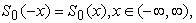we get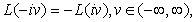where as in remark 3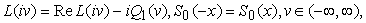and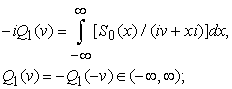we obtain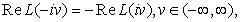But ()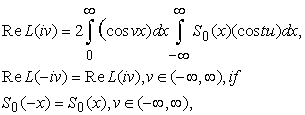and the only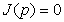function is odd and even simultaneously, we get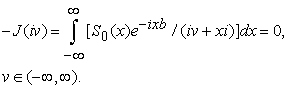### References

  Andrey Pavlov V. A new inversion formula for Laplace transforms and the notion of evenness. Hor Reser. Publ., Univer. Journ. of Appl. Mathem. USA, 2014, 2, no. 3, pp. 148-152. (http://www.hrpub.org)In article  Andrey Pavlov V. The new inversion of Laplace transforms. David Pub. Journ. of Mathem. and Syst. Scien., USA, 2014, no 4, pp. 197-201.(http://www.davidpublishing.org)In article  Pavlov A.V. Disciplines with priority to the short requirements and the identical service. Ed.of FGBOU MGTU MIREA (MIREA), numb. of gov. reg. 0321401014 from apr. 17, 2014 (http://inforeg.ru), Moscow, 2014, ISBN 978-5-7339-1261-5, 119 p.In article  Pavlov A.V. Reliable prognostication of the functions in the form of Laplace or Fourier transformations. Bulletin MGTU MIREA (77-57811 no. 180414), Moscow, 2014, 3, no. 2 (June), 78-85 pp. (http://www.mirea.ru)In article  G.M.Fihtengoltz. The course of differential and integral calculus. (In Russian). Nauka. Moscow, 1969, v. II, -800 pp.In article  Lavrentev M.A., Shabat B.V. Methods of the theory of function of complex variable. Nauka. Moscow, 1987, 544 p.In article  Kolmogorov A.N., Fomin C.V. The elements of the functions theory and the functional analysis. Nauka. Moscow, 1 976, 44 p.In article